Question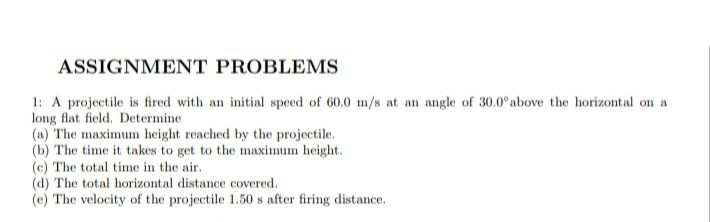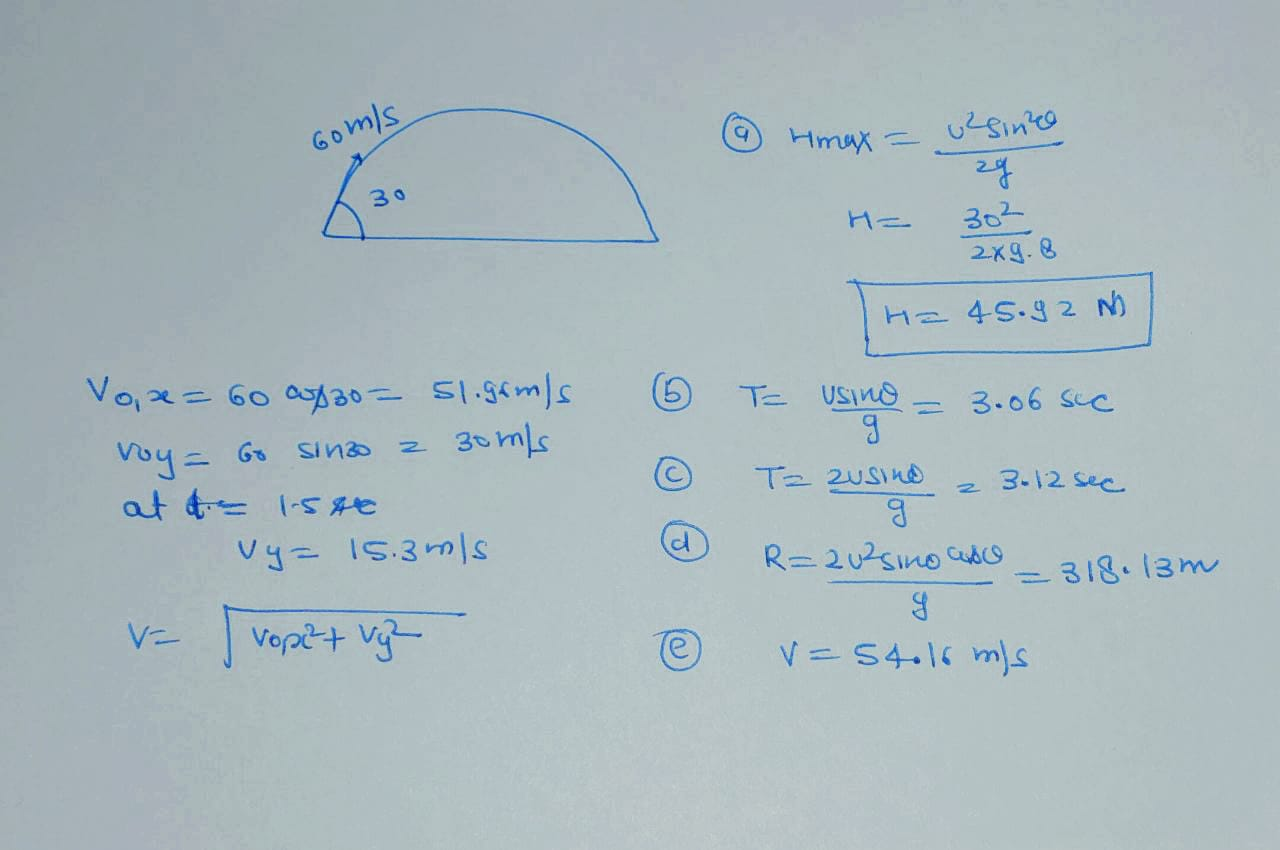#### Earn Coins

Coins can be redeemed for fabulous gifts.

Similar Homework Help Questions
• ### A projectile is fired with an initial speed of 20. m/s at an angle of 42.2°...

A projectile is fired with an initial speed of 20. m/s at an angle of 42.2° above the horizontal on a long flat firing range. Determine, a) the maximum height reached by the projectile, b) the total time in the air, c) the total horizontal distance covered (that is, the range), d) the speed of the projectile 1.50 s after firing. e) direction of the speed at 1.50 s.

• ### A projectile is fired with an initial speed of 37.4 m/s at an angle of 45.0...

A projectile is fired with an initial speed of 37.4 m/s at an angle of 45.0 ° above the horizontal on a long flat firing range.Part ADetermine the maximum height reached by the projectile. Express your answer using three significant figures and include the appropriate units. Part B Determine the total time in the air Part C Determine the total horizontal distance covered (that is, the range).

• ### A projectile is fired with an initial speed of 36.2m/s at an angle of 41.1 degrees...

A projectile is fired with an initial speed of 36.2m/s at an angle of 41.1 degrees above the horizontal on a long flat firing range. determine maxiumum height reached by projectile total time in the air determine the range speed of the projectile 1.80s after firing

• ### Determine the total time in the ar Constants A projectile is fired with an initial speed...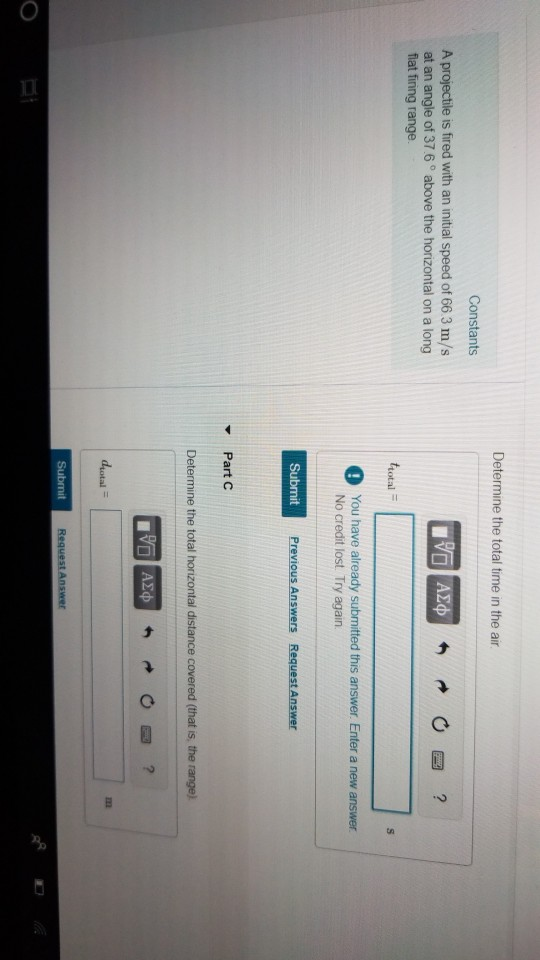Determine the total time in the ar Constants A projectile is fired with an initial speed of 66 3 m/s at an angle of 37 6 ° above the horizontal on a long total O You have already submitted this answer. Enter a new answer No credit lost. Try again Submit ▼ Part C Determine the total horizontal distance covered (that is, the range) dyotal Constants Part D A projectile is fired with an initial speed of 66.3 m/s at...

• ### A cannonball is fired from ground level at an angle of 60.0 degrees with the ground at a speed of 72.0 m/s

A cannonball is fired from ground level at an angle of 60.0 degrees with the ground at a speed of 72.0 m/s. What is the initial velocity along the x-axis?What is the initial velocity along the y-axis?What is the total time in the the air if the cannonball impacts the ground at the same vertical height as the cannonball left the cannon?What maximum height does the cannonball reach (altitude)?What is the displacement along the horizontal (range)?What is the velocity upon...

• ### A projectile is fired with an initial speed of 62.2 m/s at an angle of 43.4...

A projectile is fired with an initial speed of 62.2 m/s at an angle of 43.4 ∘ above the horizontal on a long flat firing range.Determine the direction of the projectile 1.32 s after firing.

• ### A projectile is fired with Aprojectile is fired with an initial speed of 37.9 m/s at...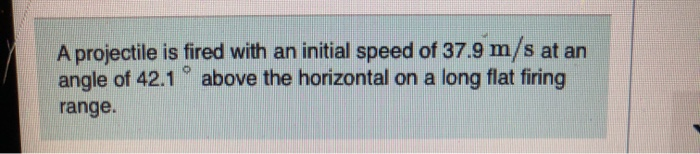A projectile is fired with Aprojectile is fired with an initial speed of 37.9 m/s at an angle of 42.1 above the horizontal on a long flat firing range. Part B Determine the total time in the air. Express your answer using three significant figures and include the appropriate units. HA ? trotal 5.081 Submit Previous Answers Request Answer xIncorrect; Try Again; 8 attempts remaining Review your calculations; you may have made a rounding error or used the wrong number...

• ### A 60.0-kg projectile is fired at an angle of 30.0° above the horizontal with an initial...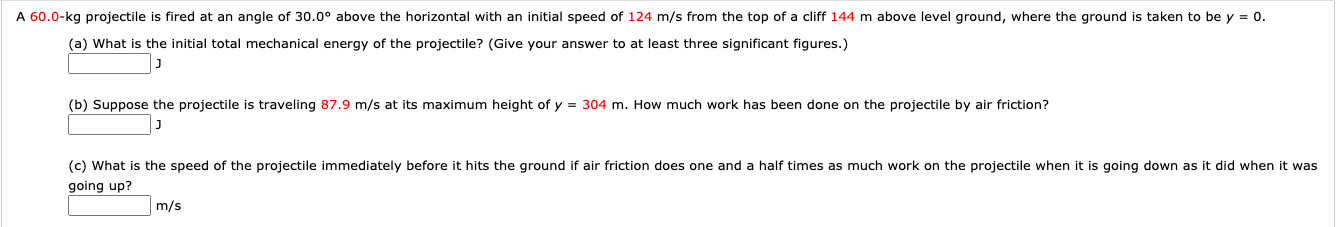A 60.0-kg projectile is fired at an angle of 30.0° above the horizontal with an initial speed of 124 m/s from the top of a cliff 144 m above level ground, where the ground is taken to be y = 0. (a) What is the initial total mechanical energy of the projectile? (Give your answer to at least three significant figures.) (b) Suppose the projectile is traveling 87.9 m/s at its maximum height of y = 304 m. How much...

• ### With steps please A projectile is fired from ground level with an initial speed of 55.6...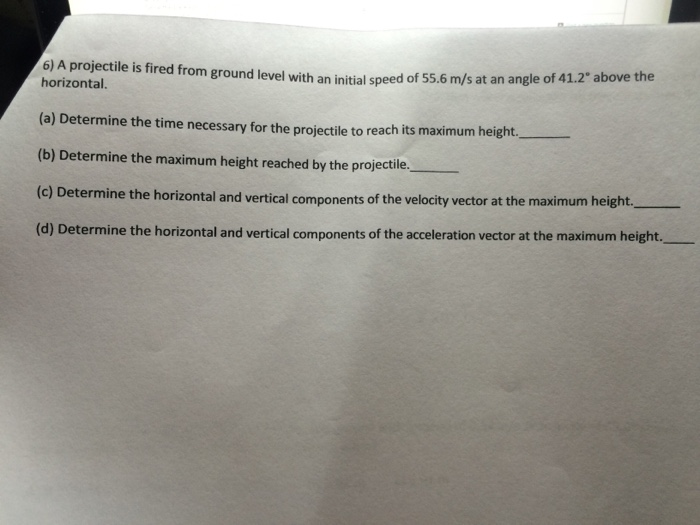With steps please A projectile is fired from ground level with an initial speed of 55.6 m/s at an angle of 41.2 above the horizontal. (a) Determine the time necessary for the projectile to reach its maximum height. (b) Determine the maximum height reached by the projectile. (c) Determine the horizontal and vertical components of the velocity vector at the maximum height. (d) Determine the horizontal and vertical components of the acceleration vector at the maximum height.